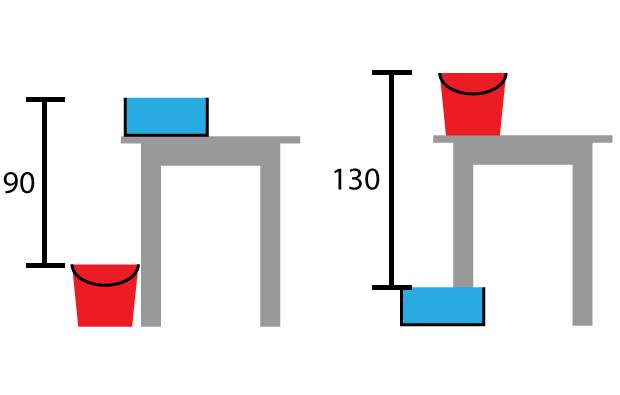# Blog

## A fish tank on the table

By

,

Jenny had a short, wide fish tank. She wanted to change the water, so she put the fish tank on a table and put a tall bucket on the floor right underneath. She was about to get a hose and siphon the water into the bucket, but before she started, she did some measuring. It was 90 centimetres from the top of the bucket to the top of the fish tank.

When she wanted to refill the tank, she swapped the set up. She put the fish tank on the ground and the bucket on the table. This time she noticed that her hose was too short – and when she measured, it was 130 centimetres from the top of the tank to the top of the bucket.

How tall was Jenny’s table?You might want to draw some diagrams to help you think through this brainteaser.

In this brainteaser, there are three objects that we don’t know the height of: the fish tank, bucket and table. The question only asks for the height of the table, and provides two measurements (90 centimetres and 130 centimetres).

Time to turn the information into equations. In the first part of the brainteaser, the top of the fish tank is one tank and one table above the floor. But the hose stretches a slightly shorter distance – one tank and one table, minus a bucket, equals 90 centimetres.
This can be written as:
Table + tank – bucket = 90 centimetres

In the second part, the tank and bucket are swapped, and the measurement is different. One bucket and one table, minus a tank equals 130 centimetres.
This can be written as:
Table + bucket – tank = 130 centimetres

If you know how to, you can use algebra to solve the question from here. Another good way to attack the problem is by estimating one number and trying it out.

### A zero estimate

Let’s assume the fish tank is 0 centimetres tall. It’s not realistic, but it makes the measurements a lot easier.

The first measurement becomes:
Table – bucket = 90 centimetres

The second measurement becomes:
Table + bucket = 130 centimetres

The difference between the table and the measurement are the same in each equation (one bucket height). So even though we don’t know how tall the bucket is, we can work out that the height of the table is precisely halfway between the two measurements. So the table is 110 centimetres tall (and the bucket is 20 centimetres tall).

### A more realistic estimate

Time to try some more reasonable numbers. Let’s start with a 30 centimetre fish tank.

The first measurement becomes:
Table + 30 centimetres (tank) – bucket = 90 centimetres
That means, table – bucket = 90 centimetres – 30 centimetres (tank) = 60 centimetres

The second measurement becomes:
Table + bucket – 30 centimetres (tank) = 130 centimetres
That means, table + bucket = 130 centimetres + 30 centimetres (tank) = 160 centimetres

The precise midpoint between 60 and 160 is, once again, 110 centimetres. In fact, no matter how tall you make the fish tank, the table will be 110 centimetres tall! And you’ll also find the bucket is always 20 centimetres taller than the tank.

Good thing you only need to calculate the height of the table!

### A different way of looking at the problem

Here’s another way of solving the problem. It is just a picture, with no words. Can you work out what’s going on?For more brainteasers and puzzles for kids, subscribe to Double Helix magazine!Categories:

## One response

1.Martin Towell

If you want to use algebra all the way:

Let T = Table, F = Fish Tank, B = Bucket
(A) T + F – B = 90
(B) T + B – F = 130

Make T the subject
(A’) T = 90 – F + B
(B’) T = 130 – B + F

Combine the equations and simplify
(A’) = (B’)
(C) 90 – F + B = 130 – B + F
(D) 2B – 2F = 40
(E) B – F = 20 (as stated in the post, the Bucket is 20cm taller than the Fish Tank)

Substitute (E) into (B) and simplify
(F) T + 20 = 130
(G) T = 110 (The height of the Table)

This site uses Akismet to reduce spam. Learn how your comment data is processed.

By posting a comment you are agreeing to the Double Helix commenting guidelines.